In very compact sources, in which the radiation energy density is comparable to the magnetic energy density, inverse Compton scattering will cause additional electron energy losses. For a homogeneous isotropic source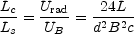(13.16)

where Lc is the power radiated by inverse Compton scattering, Ls ~ 4R2 Smc is the radio power radiated by synchrotron emission, Urad = 3L / 4 d2 c is the energy density of the radiation field, UB = B2 / 8is the energy density of the magnetic field, R is the distance to the source,the angular size, and d = Ris the source diameter. Then, from Equation (13.16), recognizing that Sm /22 is proportional to the peak brightness temperature, Tm, and including the effect of second-order scattering, we have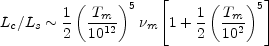(13.17)

wherem is the spectral upper cutoff frequency. Takingm ~ 100 GHz, when T < 1011, Lc / Ls << 1, inverse Compton scattering is not important. But when T > 1012 K, the second-order term becomes important, Lc / Ls ~ (Tm / 1012)10, and inverse Compton losses become catastrophic. The exact value of the peak brightness temperature which corresponds to the case where Lc ~ Lc is somewhat dependent on the specific geometry, the value of p, and the spectral cutoff frequency,m, but the strong dependence of Lc / Ls on Tm means that the maximum brightness temperature, Tm, cannot significantly exceed 1012 K, independent of wavelength. This places a lower limit to the angular size of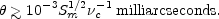(13.18)

Observations show that the peak brightness temperature of compact radio sources measured by VLBI is almost always in the range of 1011 to 1012 K. Thus, the angular size of an opaque source can be estimated from the peak flux density, Sm, and the self-absorption cutoff frequency,c to give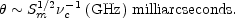(13.19)

The observed angular size is generally in good agreement with that expected from Equation (13.19) and the measured peak flux density and cutoff frequency, and there is no evidence that the peak brightness temperature ever exceeds 1012 K. This is strong evidence that the compact radio sources indeed radiate by the synchrotron process, and that the radio emission is limited by inverse Compton cooling.

The inverse Compton scattered flux density, Sc, at an energy E is given by Marscher (1983) as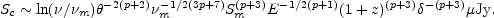(13.20)

wherem is the upper cutoff frequency of the synchrotron radiation spectrum.

Near the E = 1 keV band of the Einstein Observatory, this becomes (Biermann and Zensus 1984)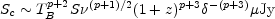(13.21)

where the effective brightness temperature, TB, is approximately22 S / 1.22 when TB is expressed in units of 1012 K.

Observations at millimeter wavelengths, where the effect of self-absorption is small, do indeed show a correlation between measured radio and X-ray flux density in the sense expected if the X-ray emission is due to inverse Compton scattering from the radio photons (Owen et al. 1981).